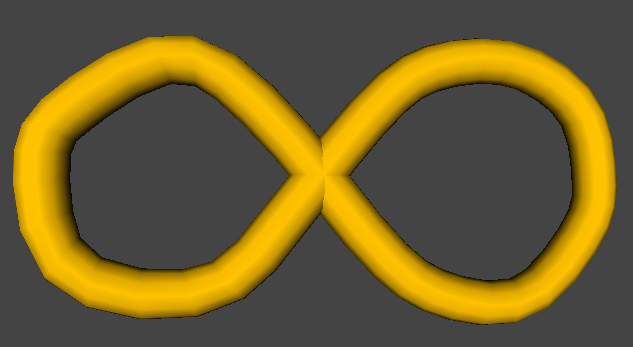# InfinityAn alternate elective after Algebra 2
by Henri Picciotto

This is a course I taught at the Urban School of San Francisco every other year from 1991 to 2011.

## Course Description

We open the course with a discussion of ancient and modern paradoxes involving infinity, and students are introduced to Georg Cantor's discoveries. Students are expected to understand and be able to reproduce his key arguments about infinite sets, including some subtle proofs by contradiction.

We explore various sequences and series, including especially the Fibonacci sequence. Much of this work requires the use of proof by mathematical induction, which students are expected to master.

Students use computers to explore dynamical systems, and thus they are introduced to chaos, a new multi-disciplinary mathematical science. In this context, we pay special attention to the iteration of the logistic function, a mathematical model of population dynamics. Students write a report about the underlying mathematics.

We explore fractal geometry through students programming their own images and calculating fractal dimensions. The course ends with an introduction to dynamical systems in the complex number plane, including the study of Julia sets and of the Mandelbrot set, one of the most spectacular discoveries of contemporary mathematics.

We make connections to literature, to philosophy, to the history of math, to science, and to other branches of math, including some review of algebra throughout the course. Iteration and recursion are concepts from mathematics and computer science that tie many of these topics together, and allow us to get a mathematical handle on infinity.

## Resources

A Teacher's Guide
Unit 1: Different-Sized Infinities
(Set Theory and Proof by Contradiction)
Galileo's Dialogue on Infinity
Worksheets
Slumber Theory
Decimals and Fractions
Irrational Numbers
Unit 2: Chaos (Dynamical Systems)
Prerequisite: Iterating Functions
Worksheets (PDF)
GeoGebra:
Iterating f(x)=rx(1-x) (in two representations)
Bifurcation Graph for f(x)=rx(1-x)
Parisa Safa's Python program, useful for the project on p. 8
(an alternative to the GeoGebra approach described on p. 9)
Unit 3: Stairway to Infinity
(Proof by Mathematical Induction)
Worksheets (PDF)
Sum of Cubes
Unit 4: Fractals (Recursion)
Some student-created fractal images (2007)
The Turtle and the Mouse (1997)
Lewis Carroll's "Two-Part Invention"

For computer programming, especially recursion, we used the Boxer "computational medium", later replacing it with the programming language Snap!Also on this site, another high school math elective: Space